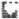`COMPUTE! ISSUE 9 / FEBRUARY 1981 / PAGE 42`

 Stat Lab A. Wachtel The 2k Experimental design is a method to determine the effect of a number of parameters which influence the outcome of a process, as well as their interactions. The main effects are considered independent from each other and orthogonal. For example, we wish to determine the effects of temperature, pressure, and agitation on the yield of a chemical reaction, or we wish to determine the number of sales per month as a function of product quality, packaging, and the amount of advertising. Combining each of the three parameters in all possible ways, we get 23 or 8 figures for yield or sales which are entered as DATA. The program employs Yate's algorithm which is simply a convenient mathematical method to arrive at the results which are read from the table which is produced. Suppose A = temperature (A = high, - = low), B = pressure (B = high, - =low), and C = agitation (C = fast, - = slow or absent), the EFFECT = denote the effects of each of these conditions on yeild. Since EFFECT2/2K = mean square, this is essentially a k - way ANOVA. An estimate of the error usually obtains from the sum of mean squares of the interactions (normally low, i.e. noise). If then, we wish to determine the confidence level for some main effect, we divide its mean square by that of the error to arrive at an F value. Replication of the experiment, i.e. obtaining two inputs for each condition is much better, because then we can obtain an independent estimate of the error from the differences between the replicates. ```0 GOTO 410 10 REM 2^K EXPERIMENTRL DESIGN A. WAC HTEL PITTSBURGH, PA 15235. 20 DIM X(32), Y(32), Z(32) 30 N = 0 40 READ Y : IF Y = 9999 THEN 80 50 N = N + 1 : I = N 60 Y(I) = Y : X(I) = Y(I) 70 GOTO 40 80 DEF FNA(X) = INT (X * 1000 + 0.5)/1000 90 K = INT(LOG(N)/LOG(2) + 0.5) 100 FOR J = 1 TO K 110 FOR I = 1 TO N/2 120 Z(I) = X(2 * I) + X(2 * I - 1) 130 NEXT I 140 FOR I = N/2 + 1 TO N 150 Z(I) = X(2 * (I - N/2)) - X(2 * (I - N/2)-1) 160 NEXT I 170 FOR I = 1 TO N 180 X(I) = Z(I) 190 PRINT "" 200 NEXT I : NEXT J 210 PRINT " N"; TAB(6) "Y"; TAB(10) "VARIABLES"; TAB(27)"ESTIMATES" 220 PRINT" ---"; TAB(5)"----"; TAB(10)"---------"; TAB(27)"--------" 230 PRINT 240 FOR N = 0 TO 2^K-1 250 J = N 260 IF J = 0 THEN A\$ = " MEAN = " : D = 2^K 270 IF J<>0 THEN A\$ = "EFFECT = " : D = 2^K/2 280 FOR I = K - 1 TO 0 STEP -1 290 K(K - I) = INT(N/2^I) : N = N - K(K - I) * 2^I 300 NEXT I 310 PRINT J + 1; TAB(4)Y(J + 1); TAB(10); 320 FOR I = K TO 1 STEP -1 330 IF K(I) = 0 THEN B\$ = " -" 340 IF K(I) = 1 THEN B\$ = " " + CHR\$(K - I + 65) 350 PRINTB\$; 360 NEXT I 370 PRINT TAB(21) A\$; TAB(30) FNA(X(J + 1)/D) 380 N = J 390 NEXT N 400 GOTO 540 410 PRINT"" 420 PRINT "THIS PROGRHM FINDS THE MAIN AND INTER-" 430 PRINT "ACTION EFFECTS OF K VARIABLES A, B, . . ." 440 PRINT "IN ALL COMBINATIONS BY YATE'S ALGORITHM" 450 PRINT" USE LINE 1 AND" 460 PRINT "ANY LINES UP TO 19 TO ENTER N DATA," 470 PRINT "FOLLOWED BY 9999. N IS ALWAYS 2^K. " 480 PRINT "(16 DATA (K = 4) WILL FIT ON THE SCREEN) 490 PRINT 500 PRINT "THE DATA CORRESPOND TO THE OBSERVATIONS" 510 PRINT "OBTAINED WITH THE VARIABLES HIGH (OR" 520 PRINT "PRESENT) = 'A, B, . .' OR LOW (OR ABSENT) = '-'." 530 PRINT "TO REGAIN INSTRUCTIONS, TYPE RUN 410." 540 END READY. ```